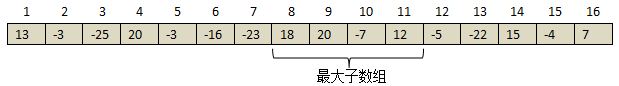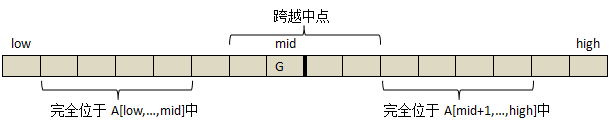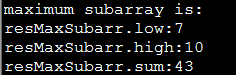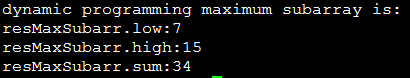# 2.问题变换# 3.使用分治策略求解最大子数组

（1）完全位于子数组A[low,…,mid]中，因此 l o w ≤ i ≤ j ≤ m i d low\leq i\leq j\leq mid
（2）完全位于子数组A[mid+1,…,high]中，因此 m i d < i ≤ j ≤ h i g h mid< i\leq j\leq high
（3）跨越了中点，因此A[low,…,mid]中，因此 l o w ≤ i ≤ m i d < j ≤ m i d low\leq i\leq mid<j\leq midFIND-MAX-CROSSING-SUBARRAY(A,low,mid,high)
left-sum=-∞
sum=0
for i=mid downto low
sum=sum+A[i]
if sum>left-sum
left-sum=sum
max-left=i
right-sum=-∞
sum=0
for j=mid+1 to high
sum=sum+A[j]
if sum>right-sum
right-sum=sum
max-right=j
return (max-left,max-right,left-sum+right-sum)


FIND-MAXIMUM-SUBARRAY(A,low,high)
if high==low
return (low,high,A[low]) //base case:only one element
else mid=⌊(low+high)/2⌋
(left-low,left-high,left-sum)=FIND-MAXIMUM-SUBARRAY(A,low,mid)
(right-low,right-high,right-sum)=FIND-MAXIMUM-SUBARRAY(A,mid+1,high)
(cross-low,cross-high,cross-sum)=FIND-MAX-CROSSING-SUBARRAY(A,low,mid,high)
if left-sum>right-sum and left-sum>cross-sum
return (left-low,left-high,left-sum)
else if right-sum>left-sum and right-sum>cross-sum
return (right-low,right-high,right-sum)
else return (cross-low,cross-high,cross-sum)


# 4.分治策略求解复杂度分析

T ( 1 ) = O ( 1 ) T(1)=O(1)

T ( n ) = 2 T ( n / 2 ) + O ( n ) T(n)=2T(n/2)+O(n)

T ( n ) = { O ( 1 ) , n = 1 2 T ( n / 2 ) + O ( n ) , n > 1 T(n)=\left\{ \begin{aligned} O(1) \hspace{2.4cm}, n=1\\ 2T(n/2)+O(n),n>1\end{aligned}\right.

# 5.示例代码

#include <iostream>

using namespace std;

struct MaximumSubarray{
int low;
int high;
int sum;
};

/******************************
func:求跨越中点的最大子数组
para:A:数组;low:左下标;mid:中下标;high:右下标
******************************/
MaximumSubarray findMaxCrossingSubarr(int A[],int low,int mid,int high){
int left_sum=0x80000000;//最小负数
int right_sum=0x80000000;//最小负数
int max_left=0;
int max_right=0;
int sum=0;
MaximumSubarray maxSubarr={0};

for(int i=mid;i>=low;--i){
sum+=A[i];
if(sum>left_sum){
left_sum=sum;
max_left=i;
}
}
sum=0;
for(int i=mid+1;i<=high;++i){
sum+=A[i];
if(sum>right_sum){
right_sum=sum;
max_right=i;
}
}
maxSubarr.low=max_left;
maxSubarr.high=max_right;
maxSubarr.sum=left_sum+right_sum;
return maxSubarr;
}

/***********************************
func:求给定数组的最大子数组
para:A:数组;low:左下标;high:右下标
***********************************/
MaximumSubarray findMaxSubarr(int A[],int low,int high){
MaximumSubarray maxSubarrLeft={0};
MaximumSubarray maxSubarrRight={0};
MaximumSubarray maxSubarrCross={0};
if(high==low){   		//特殊情况：只有一个元素
maxSubarrLeft.low=low;
maxSubarrLeft.high=high;
maxSubarrLeft.sum=A[low];
return maxSubarrLeft;
}
int mid=(low+high)/2;
maxSubarrLeft=findMaxSubarr(A,low,mid);
maxSubarrRight=findMaxSubarr(A,mid+1,high);
maxSubarrCross=findMaxCrossingSubarr(A,low,mid,high);
if(maxSubarrLeft.sum>maxSubarrRight.sum&&maxSubarrLeft.sum>maxSubarrCross.sum)
return maxSubarrLeft;
else if(maxSubarrRight.sum>maxSubarrLeft.sum&& maxSubarrRight.sum>maxSubarrCross.sum)
return maxSubarrRight;
else return maxSubarrCross;
}

int main(int argc,char* argv[]){
int A={13,-3,-25,20,-3,-16,-23,18,20,-7,12,-5,-22,15,-4,7};
MaximumSubarray resMaxSubarr=findMaxSubarr(A,0,15);
cout<<"maximum subarray is:"<<endl;
cout<<"resMaxSubarr.low:"<<resMaxSubarr.low<<endl;
cout<<"resMaxSubarr.high:"<<resMaxSubarr.high<<endl;
cout<<"resMaxSubarr.sum:"<<resMaxSubarr.sum<<endl;
return 0;
}# 6.动态规划法求解

## 6.2 动态规划法求解过程描述

m a x ( 0 , i + 1 ) = m a x { m a x ( 0 , i ) , s u m ( j , i ) + A [ i + 1 ] } , 0 ≤ j ≤ i max(0,i+1)=max \{max(0,i),sum(j,i)+A[i+1]\}, 0\leq j\leq i

## 6.3 伪代码

FIND-MAX-ARRAY(A,low,high)
if high==low
return (low,high,A[low])
max-left=low
max-right=high
sum=A[high]
tmp-sum=A[high]
for i=high-1 downto low
tmp-sum+=A[i]
if tmp-sum>sum
sum=tmp-sum
max-left=i
return (max-left,max-right,sum)


FIND-MAXIMUM-SUBARRAY(A,low,high)
if high==low
return (low,high,A[low])
max-left=low
max-right=low
sum=A[low]
for i=low+1 to high
(new-left,new-right,new-sum)=FIND-MAX-ARRAY(A,low,i)
if(new-sum>sum)
(max-left,max-right,max-sum)=(new-left,new-right,new-sum)
return (max-left,max-right,max-sum)


## 6.4 示例代码

//dynamic programming method
/**********************************************
func:获取以high为起始下标向左至low下标的最大子数组
para:A:数组;low:起始下标;high:结束下标
**********************************************/
MaximumSubarray FIND_MAX_ARRAY(int A[],int low,int high){
MaximumSubarray maxSubarr={0};
if (high==low){
maxSubarr.low=low;
maxSubarr.high=high;
maxSubarr.sum=A[low];
return maxSubarr;
}
maxSubarr.low=high;
maxSubarr.high=high;
maxSubarr.sum=A[high];
int tmp_sum=A[high];
for (int i=high-1;i>=low;--i){
tmp_sum+=A[i];
if(tmp_sum>maxSubarr.sum){
maxSubarr.sum=tmp_sum;
maxSubarr.low=i;
}
}
return maxSubarr;
}

/***********************************************
func:求给定数组的最大子数组
para:A:数组;low:起始下标;high:结束下标
***********************************************/
MaximumSubarray FIND_MAXIMUM_SUBARRAY(int A[],int low,int high){
MaximumSubarray maxSubarr={0};
if (high==low){
maxSubarr.low=low;
maxSubarr.high=high;
maxSubarr.sum=A[low];
return maxSubarr;
}

maxSubarr.low=low;
maxSubarr.high=low;
maxSubarr.sum=A[low];
MaximumSubarray tmpSubarr={0};
for (int i=low+1; i<=high;++i)
tmpSubarr=FIND_MAX_ARRAY(A,low,i);
if(tmpSubarr.sum>maxSubarr.sum)
maxSubarr=tmpSubarr;
return maxSubarr;
}

int main(int argc,char* argv[]) {
int A={13,-3,-25,20,-3,-16,-23,18,20,-7,12,-5,-22,15,-4,7};
MaximumSubarray resMaxSubarr=FIND_MAXIMUM_SUBARRAY(A,0,15);
cout<<"dynamic programming maximum subarray is:"<<endl;
cout<<"resMaxSubarr.low:"<<resMaxSubarr.low<<endl;
cout<<"resMaxSubarr.high:"<<resMaxSubarr.high<<endl;
cout<<"resMaxSubarr.sum:"<<resMaxSubarr.sum<<endl;
return 0;
}# 7.扫描法

int MaxSubSum3(int *arr,int len) {
int i;
int MaxSum = 0;
int CurSum = 0;
for(i=0;i<len;i++){
CurSum += arr[i];
if(CurSum > MaxSum)
MaxSum = CurSum;
//如果累加和出现小于0的情况,
//则最大和子序列肯定不可能包含前面的元素，
//这时将累加和置0，从下个元素重新开始累加
if(CurSum < 0)
CurSum = 0;
}
return MaxSum;
}


# 参考文献

 算法导论中文版.原书第三版[M].P38-42
 动态规划：从新手到专家
【算法拾遗】三种方法求连续子数组的最大和

07-194994
05-021万+03-211234
09-26147
11-054965
01-1348
05-2247
05-2253
08-071万+
07-121357